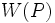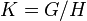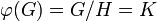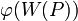# Equivalence of definitions of conjugacy functor whose normalizer generates whole group with p'-core

This article gives a proof/explanation of the equivalence of multiple definitions for the term conjugacy functor whose normalizer generates whole group with p'-core
View a complete list of pages giving proofs of equivalence of definitions

## Statement

Suppose$G$ is a group,$p$ is a prime number, and$W$ is a conjugacy functor for$G$. The following conditions are equivalent, where$P$ is any$p$-Sylow subgroup of$G$.

1.$O_{p'}(G)N_G(W(P)) = G$
2. The image of$W(P)$ in the quotient$G/O_{p'}(G)$ is a normal subgroup of$G/O_{p'}(G)$.

## Proof

### (2) implies (1)

Given: A prime$p$, a finite group$G$ such that if$K = G/O_{p'}(G)$, then for every$p$-Sylow subgroup$P$ of$G$, the image of$W(P)$ is normal in$K$.

To prove: For one (and hence every)$p$-Sylow subgroup$P$ of$G$,$\! G = O_{p'}(G)N_G(W(P))$.

Proof:

Step no. Assertion/construction Facts used Given data used Previous steps used Explanation
1 Let$H = O_{p'}(G)$, so$K = G/H$, and$\varphi:G \to G/H$ be the quotient map, with$\varphi(G) = G/H = K$. PLACEHOLDER FOR INFORMATION TO BE FILLED IN: [SHOW MORE]
2 Let$L = \varphi^{-1}(\varphi(W(P))))$. In other words,$\! L = O_{p'}(G)W(P)$.
3$L$ is normal in$G$. Fact (1)$\varphi(W(P))$ is normal in$K$. Steps (5), (6) fact-step combination direct.
4$\! W(P)$ is$p$-Sylow in$\! L$. Step (2) [SHOW MORE]
5$\! LN_G(W(P)) = G$. Fact (2) Steps (3), (4) Fact-step-combination direct.
6$\! O_{p'}(G)N_G(W(P))) = G$ Steps (2), (5) [SHOW MORE]